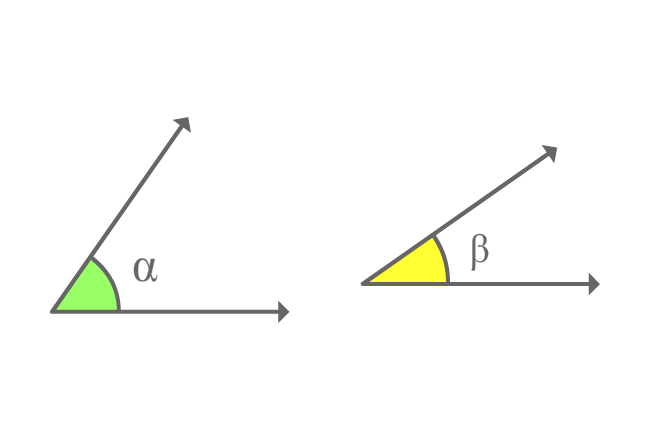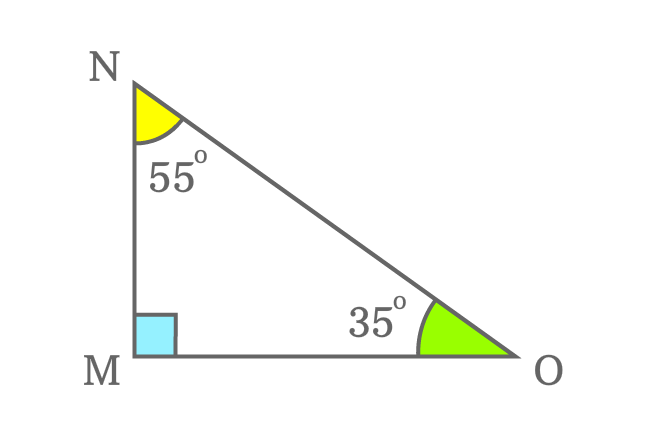# Complementary anglesThe angles whose sum equals to $90^\circ$ are called the complementary angles.

## Introduction

Complementary angles are angles basically but the sum of them represents a right angle when the angles are added to each other.

One angle is known as complement of another angle and vice-versa.

### Formula$\alpha$ and $\beta$ are two angles. The sum of them forms a right angle geometrically.

$\alpha+\beta = 90^\circ$

1. $\alpha$ is called complement of $\beta$
2. $\beta$ is called complement of $\alpha$

The angles $\alpha$ and $\beta$ are known as complementary angles.

### Example$\Delta MON$ is a right triangle.

It has three interior angles. One angle ($\angle OMN$) is right angle. The other two angles are $\angle MNO$ and $\angle NOM$.

The $\angle MNO$ is $55^\circ$ and the $\angle NOM$ is $35^\circ$. Now, add both angles.

$\angle MNO + \angle NOM$ $=$ $55^\circ + 35^\circ$

$\implies$ $\angle MNO + \angle NOM$ $=$ $90^\circ$

The sum of two angles is right angle $\Big(\dfrac{\pi}{2}\Big)$. Therefore, the angles ($\angle MNO$ and $\angle NOM$) are called as complementary angles.

The $\angle MNO$ is called as complement of the $\angle NOM$. Similarly, $\angle NOM$ is called as complement of the $\angle MNO$.

A best free mathematics education website that helps students, teachers and researchers.

###### Maths Topics

Learn each topic of the mathematics easily with understandable proofs and visual animation graphics.

###### Maths Problems

A math help place with list of solved problems with answers and worksheets on every concept for your practice.

Learn solutions

###### Subscribe us

You can get the latest updates from us by following to our official page of Math Doubts in one of your favourite social media sites.